# The multi-VAR Framework

The $$\texttt{multivar}$$ package (Fisher, 2021) is intended to provide an entry point to the multi-VAR algorithm (Fisher, Kim, Fredrickson and Pipiras, 2022). In short, multi-VAR is designed to model multivariate time series obtained from multiple individuals. This method is especially well-suited to time-series paradigms, such as intensive longitudinal data (ILD), where it is often unclear the degree to which individuals differ in terms of their dynamic processes. If individuals share little in common results from multi-VAR resemble what would be obtained from fitting separate models to each individual. If individuals are homogenous, results resemble what would be obtained from pooling the data and fitting a single model to the sample. Most importantly, if the truth lies somewhere in between - certain dynamics are shared while others are idiosyncratic - results will reflect this and provide researchers with new tools for isolating generalizable dynamics. Although this vignette is not intended to provide a thorough introduction to multi-VAR it introduces the $$\texttt{multivar}$$ package functionality.

## Load the $$\texttt{multivar}$$ Package

library("multivar")

# A Simulated multi-VAR Example

Simulated data is one way to gain insight into the problem the multi-VAR framework attempts to solve. Here, we consider the $$\texttt{dat_multivar_sim}$$ dataset included in the $$\texttt{multivar}$$ package. This dataset contains multivariate time series data for $$k = 9$$ individuals with $$d = 10$$ variables, collected at $$t = 100$$ equidistant time points. The data was generated such that each individual’s VAR(1) transition matrix has $$20\%$$ nonzero entries. This means, for example, each individual has 20 nonzero directed relationships in their data generating model. The position of non-zero elements in each individual’s transition matrix was selected randomly given the following constraints: $$2/3$$ of each individual’s paths are shared by all individuals, and $$1/3$$ are unique to each individual. For each individual, coefficient values between $$\mathcal{U}(0,1, 0.9)$$ were randomly drawn until stability conditions for the VAR model were satisfied. The underlying idea is multivariate time series arising from multiple units are often heterogeneous. These generated data reflect one example of data fitting this description.

## Plot Simulated Data

We can visualize the transition matrices for the simulated data as follows.

### Common Effects

To plot the effects common to each individual use the option $$\texttt{plot_type = "common"}$$.

plot_sim(dat_multivar_sim, plot_type = "common")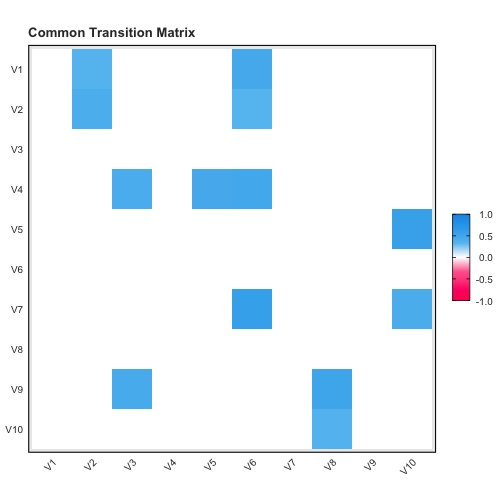plot of chunk unnamed-chunk-2

### Total Effects

To plot the total effects for each individual use the option $$\texttt{plot_type = "total"}$$.

plot_sim(dat_multivar_sim, plot_type = "total")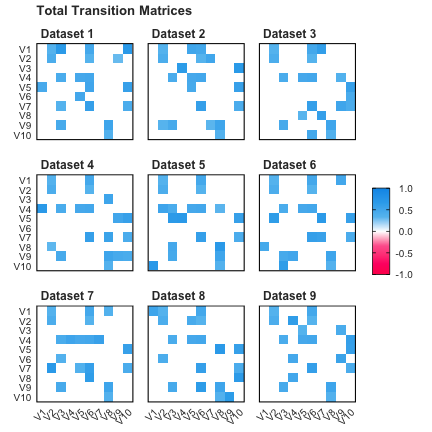plot of chunk unnamed-chunk-3

# Construct a multi-VAR Model

With data in hand we can construct a multi-VAR model. The $$\texttt{data}$$ argument should be a list containing the $$k$$ multivariate time series data from each individual. Each data matrix should be organized with variables as columns and time points as rows (e.g. $$T \times d$$). By default $$\texttt{multivar}$$ employs the adaptive LASSO penalty, which requires an initial estimate of the individual transition matrices. The default option for an initial weight matrix based on estimates from the individual-level LASSO. Additional details on these initial weights and the adaptive lasso can be found in Fisher, Kim, Fredrickson and Pipiras (2022).

model <- multivar::constructModel(data = dat_multivar_sim\$data)

# Cross-Validate

As of Version 1.0.0 the only cross-validation procedure available in $$\texttt{multivar}$$ are rolling window cross-validation (RWCV) or blocked k-folds CV (blocked). The default is blocked with 5 folds. Additional cross-validation methods for mult-VAR are currently under development and should be available soon.

fit <- multivar::cv.multivar(model)

## Plot Results

After performing cross-validation, results from the multi-VAR procedure can be visualized using the $$\texttt{plot_results()}$$ function. Below we show the estimated common and total effects matrices.

### Common Effects

plot_results(fit, plot_type = "common")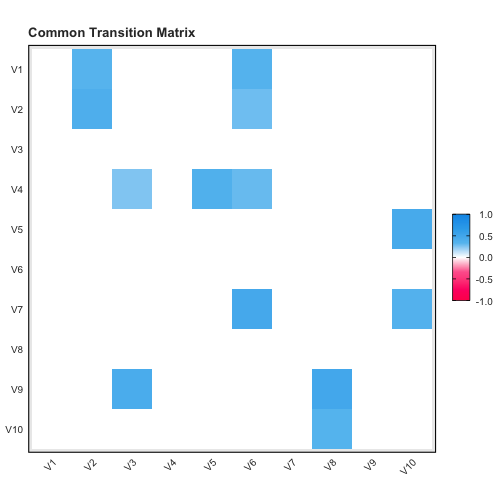plot of chunk unnamed-chunk-6

### Total Effects

plot_results(fit, plot_type = "total")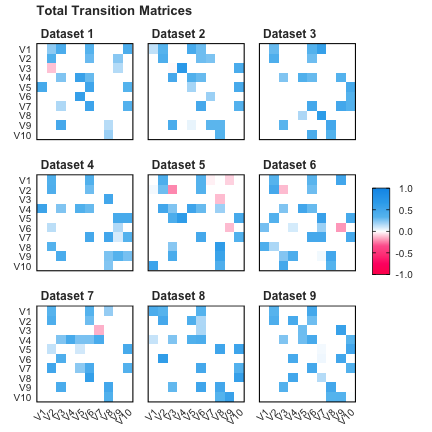plot of chunk unnamed-chunk-7

## Compare Simulated Data to Results

Finally, we can compare the results from the first three individuals to the data generating transition matrices as follows.

### Common Effects

gridExtra::grid.arrange(
plot_sim(dat_multivar_sim, plot_type = "common"),
plot_results(fit, plot_type = "common"),
ncol = 1
)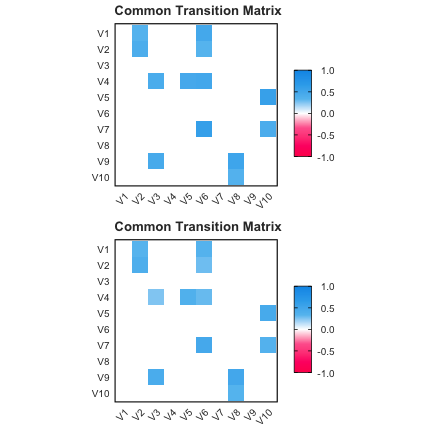plot of chunk unnamed-chunk-8

### Total Effects

gridExtra::grid.arrange(
plot_sim(dat_multivar_sim, plot_type = "total", datasets = c(1:3)),
plot_results(fit, plot_type = "total", datasets = c(1:3)),
ncol = 1
)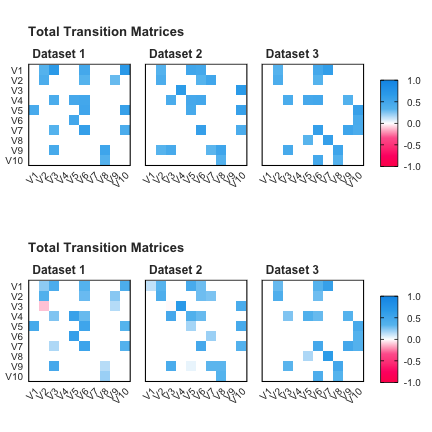plot of chunk unnamed-chunk-9